# TwoStack PDA CS 6800 Advance Theory of Computation

• Slides: 51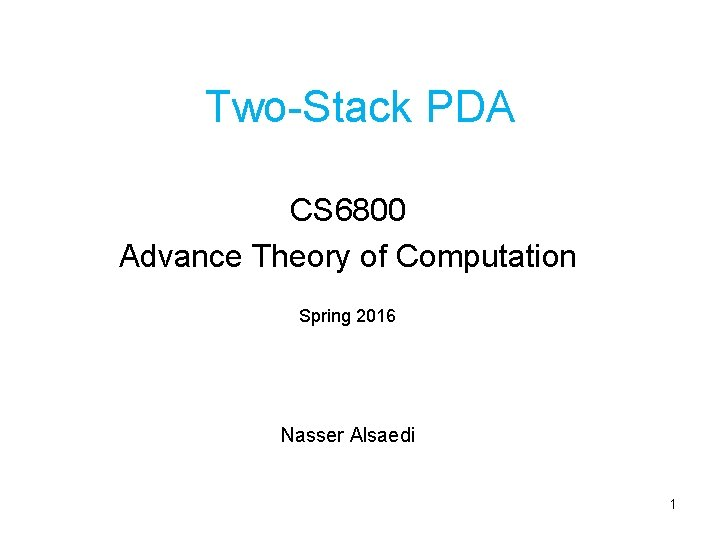Two-Stack PDA CS 6800 Advance Theory of Computation Spring 2016 Nasser Alsaedi 1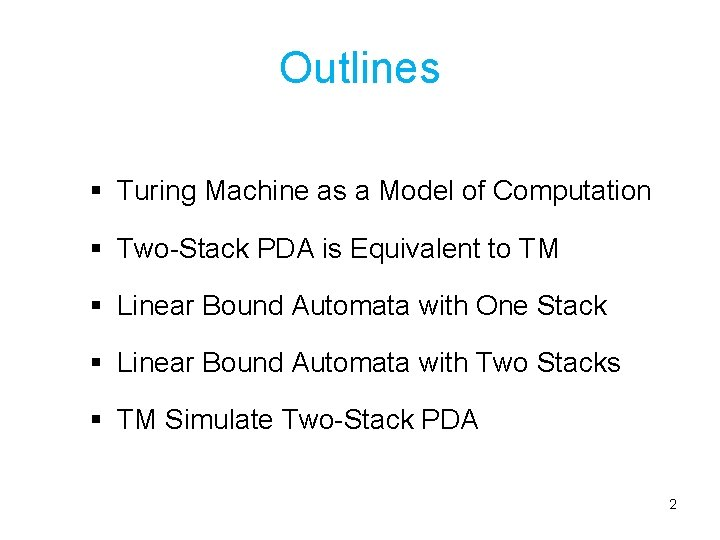Outlines § Turing Machine as a Model of Computation § Two-Stack PDA is Equivalent to TM § Linear Bound Automata with One Stack § Linear Bound Automata with Two Stacks § TM Simulate Two-Stack PDA 2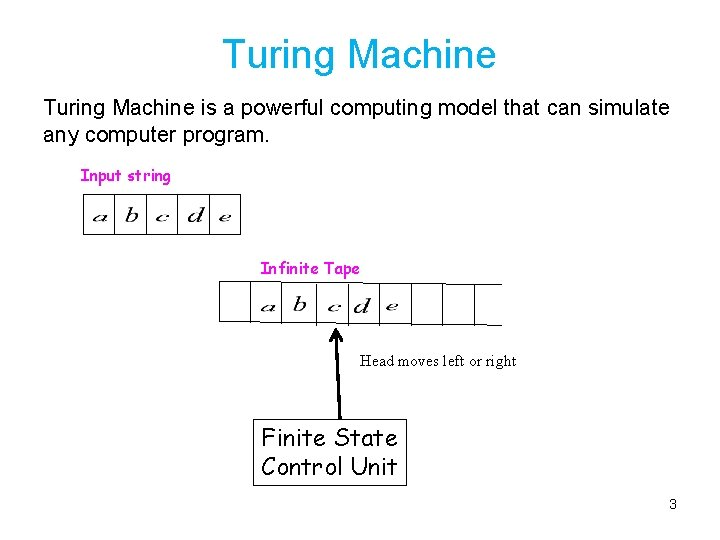Turing Machine is a powerful computing model that can simulate any computer program. Input string Infinite Tape Head moves left or right Finite State Control Unit 3The Turing Machine Hopcroft and Ullman  defined a one-tape Turing machine as sextuples (Q, Γ, ∑, , q 0, F) where: • Q is a finite set of states. • Γ is a finite set of the tape alphabet/symbols contain a special symbol B that represent a blank. • Σ is a subset of Γ – {B} called input alphabet. • = Q x Γ x {L, R} is a partial function called the transition function, where L is left shift, R is right shift. • q 0 is the initial state. • F is the set of final or accepting states. 4Turing Machine as Model of Computation § Turing Machine is a model of computation. § Equivalent versions of Turing machine have the same power as the standard Turing machine. − − Two way Turing Machine Multitrack Turing Machine Multitape Turing Machine Non-deterministic Turing Machine § Any machine that simulates any computer program can be considered as model of computation. § Undecidable Problem can be apply to any computational model (not restricted to the Turing machine). 5Two-Stack PDA Ø A Turing machine can accept languages not accepted by any PDA with one stack. Ø The strength of pushdown automata can be increased by adding additional (extra) stacks. Ø Actually, a PDA with two stacks has the same computation power as a Turing Machine. 6Two-Stack PDA Input Tape Head moves from left to write Finite state control unit Stack 1 Stack 2 7Two-Stack PDA k-Stack PDA is also called k-stack machine. Adding more than two stacks to multistack machine does not increase the power of computation. Theorem (8. 13) (Hopcroft and Ullman ): If a language L is accepted by a Turing machine, then L is accepted by a two-stack machine. 8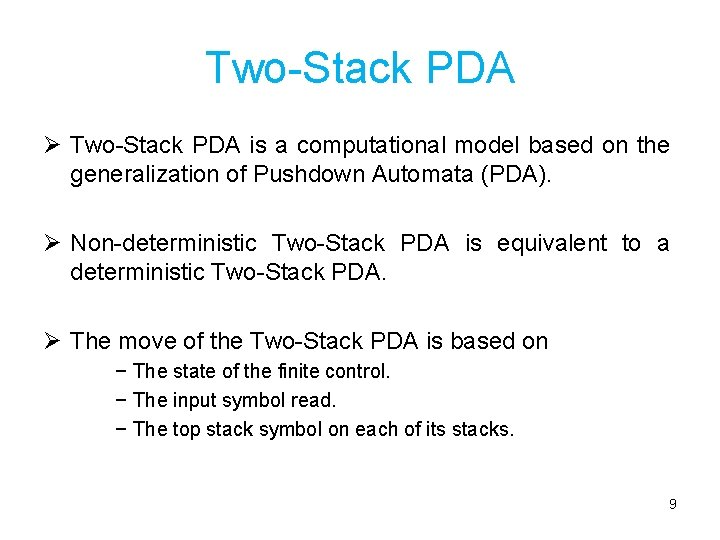Two-Stack PDA Ø Two-Stack PDA is a computational model based on the generalization of Pushdown Automata (PDA). Ø Non-deterministic Two-Stack PDA is equivalent to a deterministic Two-Stack PDA. Ø The move of the Two-Stack PDA is based on − The state of the finite control. − The input symbol read. − The top stack symbol on each of its stacks. 9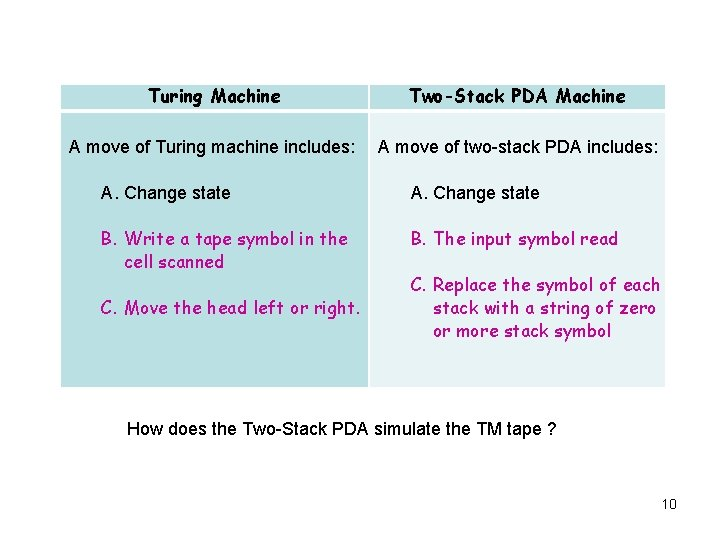Turing Machine Two-Stack PDA Machine A move of Turing machine includes: A move of two-stack PDA includes: A. Change state B. Write a tape symbol in the cell scanned B. The input symbol read C. Replace the symbol of each stack with a string of zero or more stack symbol C. Move the head left or right. How does the Two-Stack PDA simulate the TM tape ? 10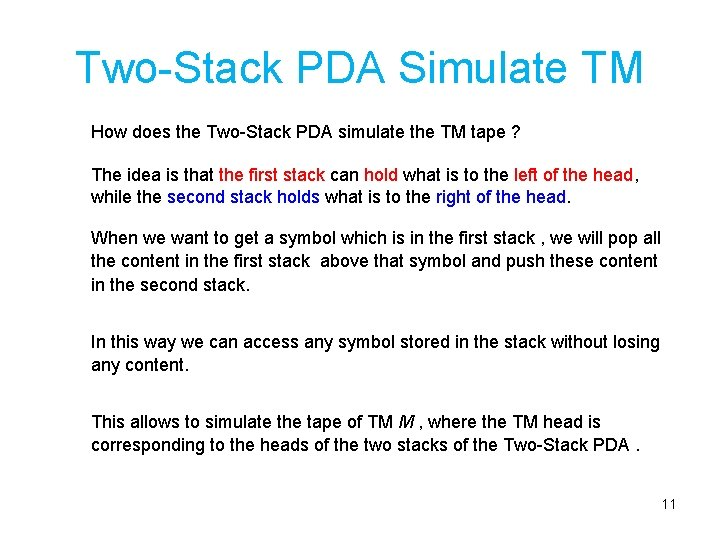Two-Stack PDA Simulate TM How does the Two-Stack PDA simulate the TM tape ? The idea is that the first stack can hold what is to the left of the head, while the second stack holds what is to the right of the head. When we want to get a symbol which is in the first stack , we will pop all the content in the first stack above that symbol and push these content in the second stack. In this way we can access any symbol stored in the stack without losing any content. This allows to simulate the tape of TM M , where the TM head is corresponding to the heads of the two stacks of the Two-Stack PDA. 11TM M Two-Stack PDA S M Tape Stack 1 Stack 2 12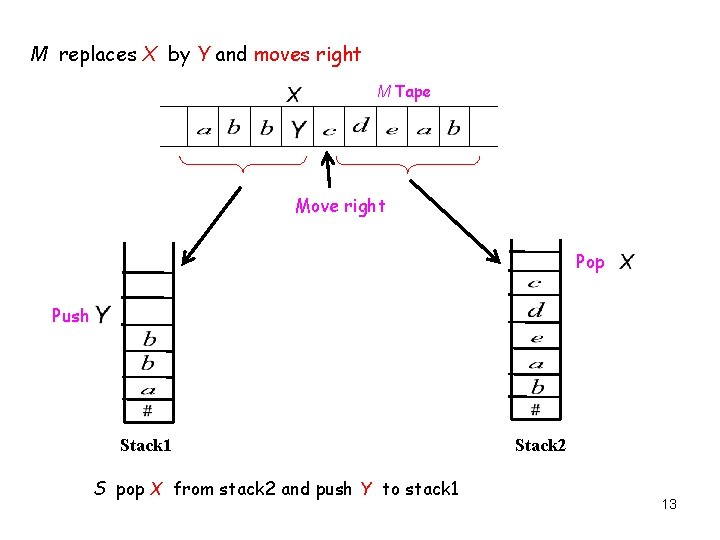M replaces X by Y and moves right M Tape Move right Pop Push Stack 1 S pop X from stack 2 and push Y to stack 1 Stack 2 13M replaces X by Y and moves left M Tape Move left push Y Pop Stack 1 Stack 2 S pop X and Z from stack 1 and push ZY to stack 2 14Pushdown Automata Sudkamp  defined A pushdown automata (PDA) as a sextuple (Q, , q 0, F), where Ø Q is a finite set of states Ø is a finite set of input symbols, called input alphabet Ø is a finite set of stack symbols, called stack alphabet Ø q 0 Q, is the start state Ø F Q, is the set of final states Ø : Q ( { }) ( { }) to the set of all subsets of Q ( { }), a (partial) transition function 15Pushdown Automata Input Tape Head moves from left to right state control Stack PDA = FSA+ memory (stack) 16PDA The transition is of the form: [qj, B] (qi, a, A), where Ø qi is the current state Ø a is the current input symbol Ø A is the current top of the stack symbol Ø qj is the new state Ø B is the new top of the stack symbol qi a A/B qj qi qi a / a A/ qj qj 17Accepting Criteria There are two criteria for string acceptance by PDA. Ø First Criteria (final state and empty stack) An input string x is accepted by the PDA if the PDA stops at a final state and the stack is empty. Otherwise, the input string is rejected. Ø Second Criteria (final state only) An input string x is accepted by the PDA if the PDA stops at a final state no matter what symbols in the stacks. Let L be a language accepted by a PDA M (Q, , q 0, F) with acceptance defined by final state. Then there is a PDA that accepts L by final state and empty stack. 18Context-Free Grammar (CFG) Productions One variable String of variables and terminals A language L is context-free if there exists a CFG G such that L = L(G). Theorem: Let L be a context-free language, then there is a PDA that accepts L. 19The language is context-free: If (n > 0) , the context-free grammar as follow: If (n 0) , the context-free grammar as follow: 20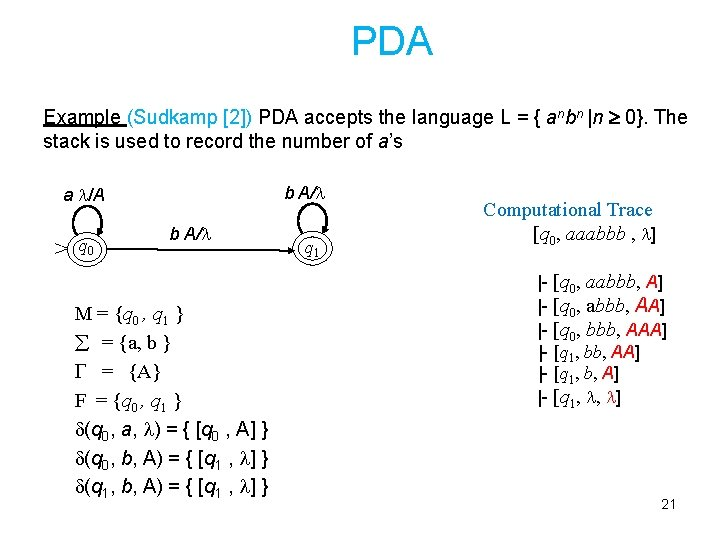PDA Example (Sudkamp ) PDA accepts the language L = { anbn |n 0}. The stack is used to record the number of a’s a /A > q 0 b A/ M = {q 0 , q 1 } = {a, b } = {A} F = {q 0 , q 1 } (q 0, a, ) = { [q 0 , A] } (q 0, b, A) = { [q 1 , ] } (q 1, b, A) = { [q 1 , ] } q 1 Computational Trace [q 0, aaabbb , ] |- [q 0, aabbb, A] |- [q 0, abbb, AA] |- [q 0, bbb, AAA] |- [q 1, bb, AA] |- [q 1, b, A] |- [q 1, , ] 21Pushdown Automata is non-deterministic PDA accepts the language L = { anbn |n 0}. a /A > q 0 b A/ q 1 OR a /A > q 0 b A/ / q 1 22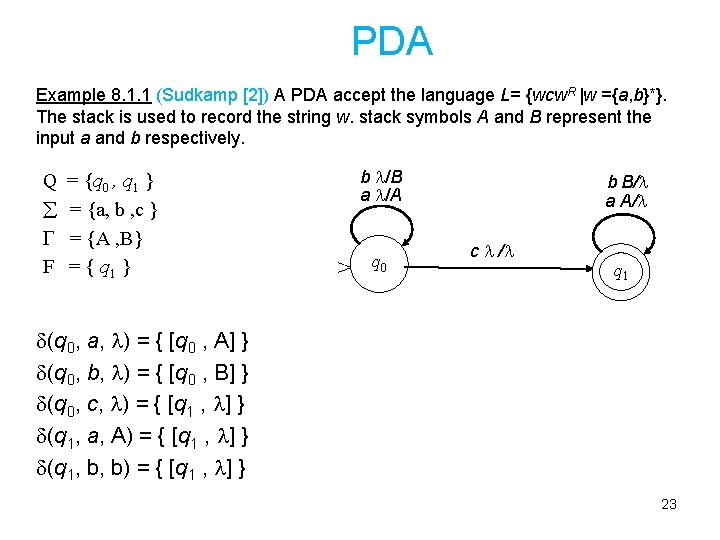PDA Example 8. 1. 1 (Sudkamp ) A PDA accept the language L= {wcw. R |w ={a, b}*}. The stack is used to record the string w. stack symbols A and B represent the input a and b respectively. Q = {q 0 , q 1 } = {a, b , c } = {A , B} F = { q 1 } b /B a /A > q 0 b B/ a A/ c / q 1 (q 0, a, ) = { [q 0 , A] } (q 0, b, ) = { [q 0 , B] } (q 0, c, ) = { [q 1 , ] } (q 1, a, A) = { [q 1 , ] } (q 1, b, b) = { [q 1 , ] } 23Computational Power of PDA Ø PDA accepts only context sensitive languages. Ø Some languages can not be accepted by PDA. Example of languages not accepted by PDA Language Machine Grammar L = { aibici | i > 0 } LBA Context sensitive L = { aibicidi | i 0 } TM Unrestricted L = { aibicidiei | i 0 } TM Unrestricted 24Context-Sensitive Grammar (CSG) Productions String of variables and terminals and A language L is context-sensitive if there exists a CSG G such that L = L(G). Theorem: Let L be a context-sensitive language, then there is a linear-bounded automata M with L(M)= L. 25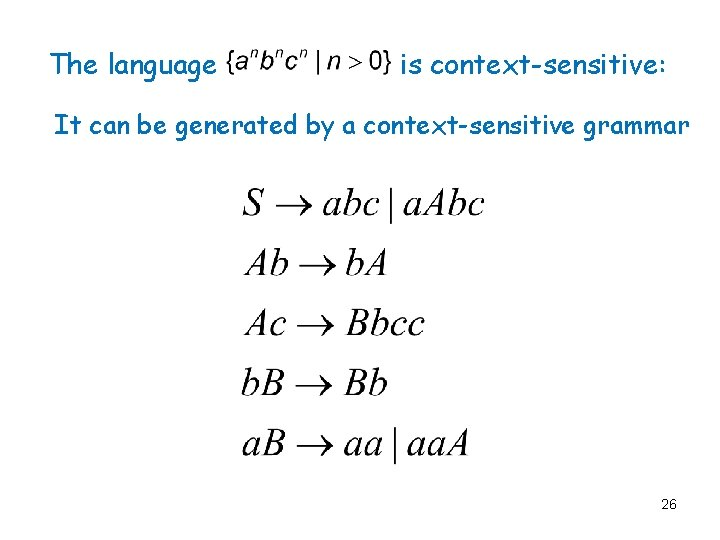The language is context-sensitive: It can be generated by a context-sensitive grammar 26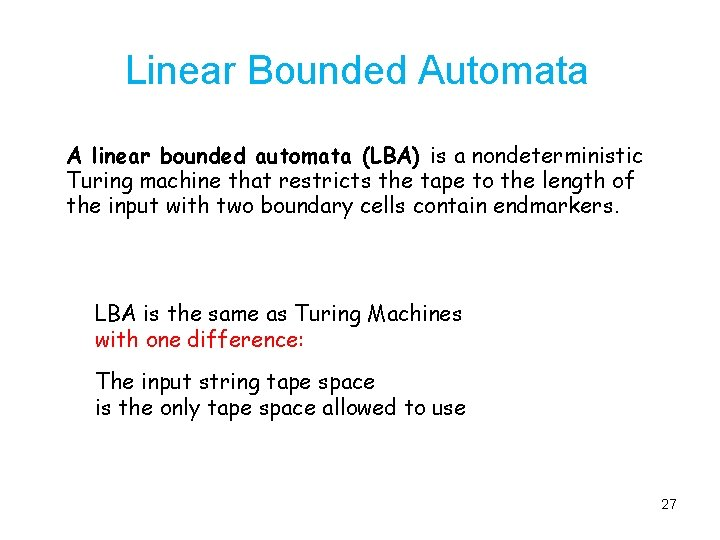Linear Bounded Automata A linear bounded automata (LBA) is a nondeterministic Turing machine that restricts the tape to the length of the input with two boundary cells contain endmarkers. LBA is the same as Turing Machines with one difference: The input string tape space is the only tape space allowed to use 27Linear Bounded Automaton (LBA) Input string Left-end marker Finite State Control Unit Right-end marker All computation is done between end markers 28Unrestricted Grammars Productions String of variables and terminals Theorem: A language L is recursively enumerable if and only if L is generated by an unrestricted grammar. 29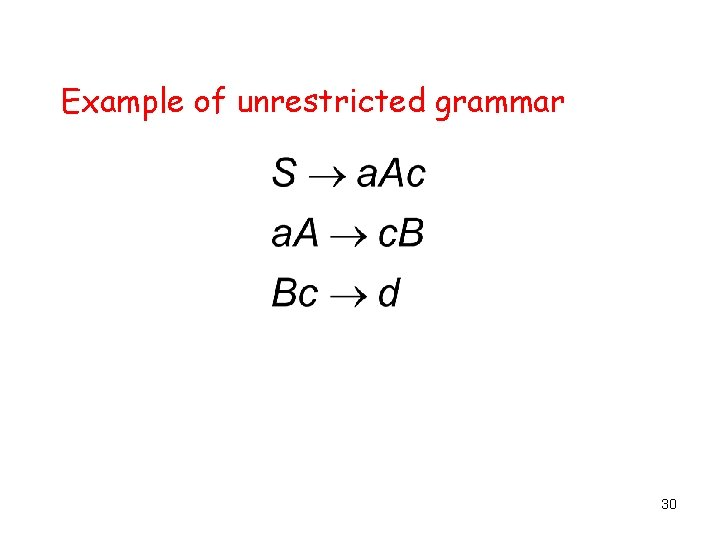Example of unrestricted grammar 30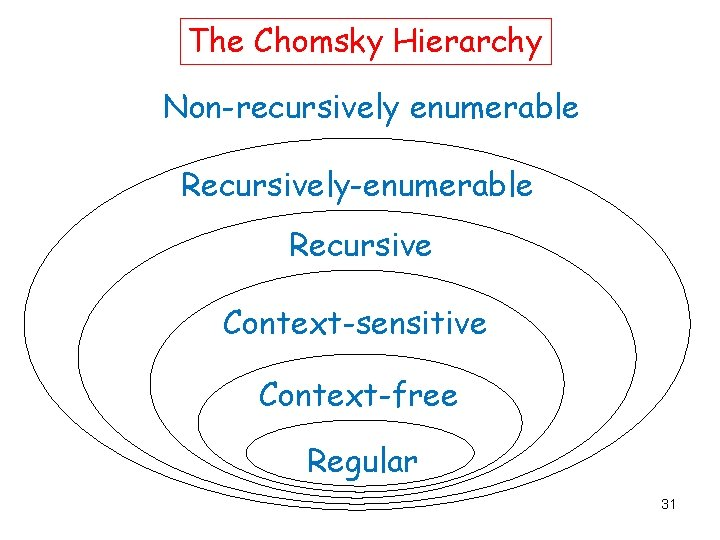The Chomsky Hierarchy Non-recursively enumerable Recursively-enumerable Recursive Context-sensitive Context-free Regular 31Two-Stack PDA Definition 8. 6. 1 Sudkamp  A two-stack PDA is a sextuple (Q, , q 0, F), where Q, , , q 0, and F are the same as in a one-stack PDA. The transition function as follow: : Q ( { }) ( { }) to the set of all subsets of Q ( { }) The two stacks of the PDA are independent. one stack: a A/ qi two stack: qj qi a A/ /B/C qj Two-Stack PDA accepts any language that accepted by a Turing Machine. 32Two-Stack PDAs Two-Stack PDA accepts the language L= { aibici | i 0 } a /A / > q 0 c / B/ b A/ /B q 1 c / B/ q 2 OR a /A / > q 0 c / B/ / / b A/ /B q 2 c / B/ q 1 b A/ /B 33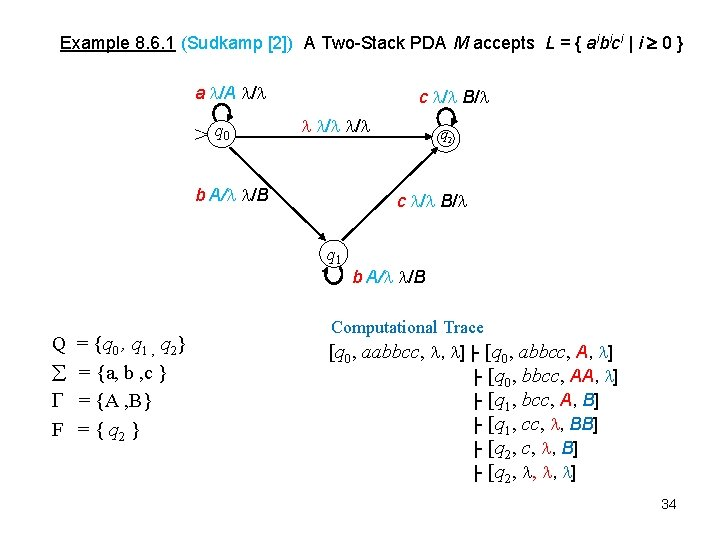Example 8. 6. 1 (Sudkamp ) A Two-Stack PDA M accepts L = { aibici | i 0 } a /A / > q 0 c / B/ / / b A/ /B c / B/ q 1 Q F = {q 0 , q 1 , q 2} = {a, b , c } = {A , B} = { q 2 } q 2 b A/ /B Computational Trace [q 0, aabbcc, , ] |- [q 0, abbcc, A, ] |- [q 0, bbcc, AA, ] |- [q 1, bcc, A, B] |- [q 1, cc, , BB] |- [q 2, c, , B] |- [q 2, , , ] 34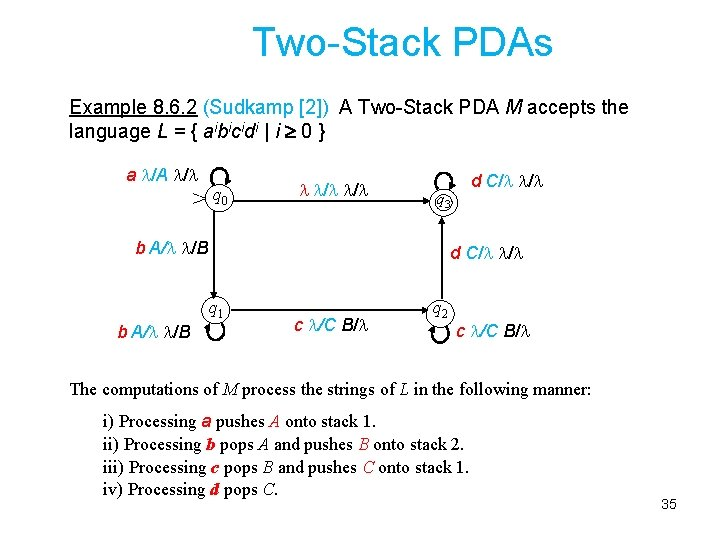Two-Stack PDAs Example 8. 6. 2 (Sudkamp ) A Two-Stack PDA M accepts the language L = { aibicidi | i 0 } a /A / > q 0 / / q 3 b A/ /B d C/ / q 1 b A/ /B d C/ / c /C B/ q 2 c /C B/ The computations of M process the strings of L in the following manner: i) Processing a pushes A onto stack 1. ii) Processing b pops A and pushes B onto stack 2. iii) Processing c pops B and pushes C onto stack 1. iv) Processing d pops C. 35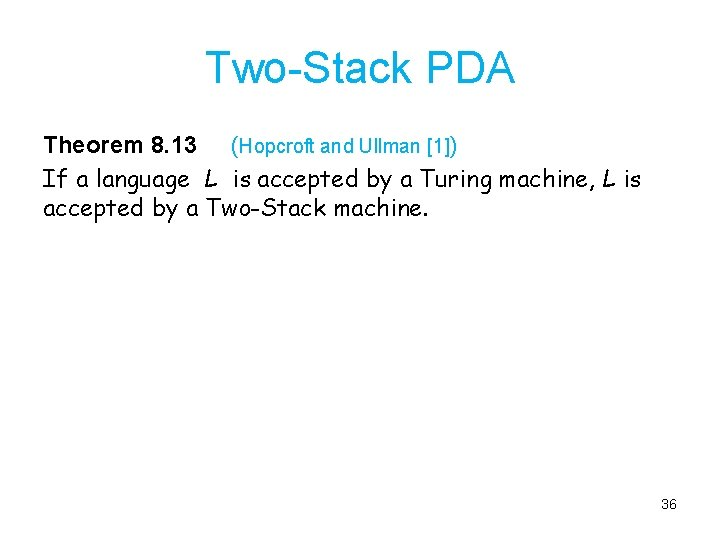Two-Stack PDA Theorem 8. 13 (Hopcroft and Ullman ) If a language L is accepted by a Turing machine, L is accepted by a Two-Stack machine. 36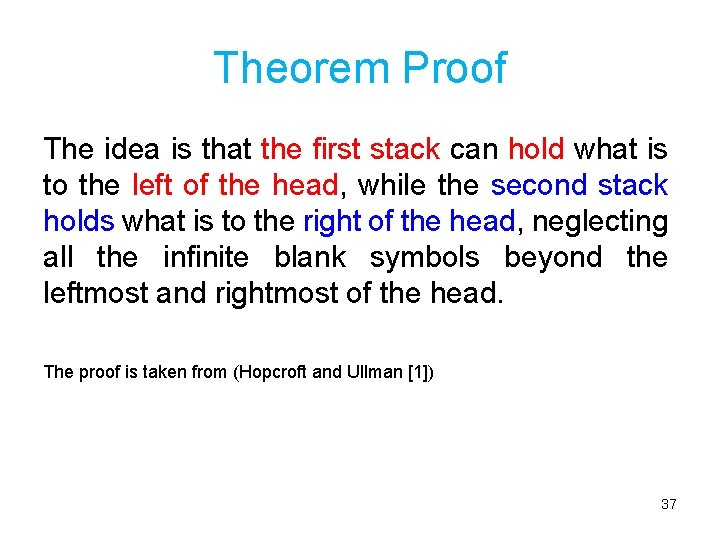Theorem Proof The idea is that the first stack can hold what is to the left of the head, while the second stack holds what is to the right of the head, neglecting all the infinite blank symbols beyond the leftmost and rightmost of the head. The proof is taken from (Hopcroft and Ullman ) 37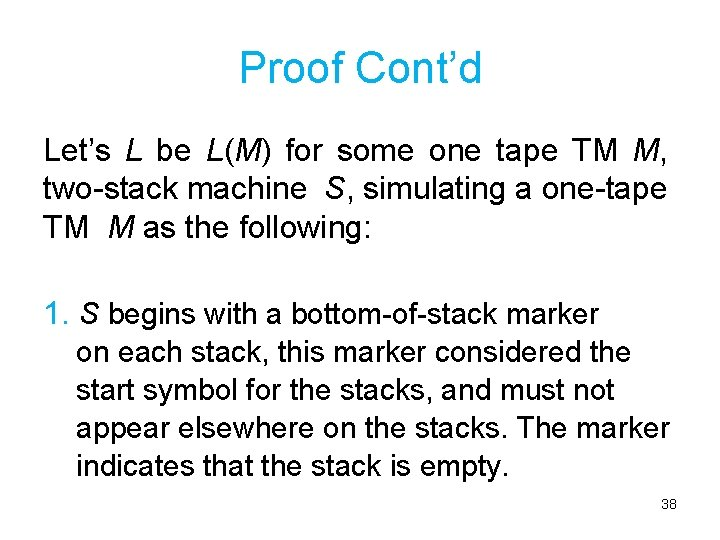Proof Cont’d Let’s L be L(M) for some one tape TM M, two-stack machine S, simulating a one-tape TM M as the following: 1. S begins with a bottom-of-stack marker on each stack, this marker considered the start symbol for the stacks, and must not appear elsewhere on the stacks. The marker indicates that the stack is empty. 38Proof Cont’d 2. Suppose that w\$ is on the input of S. S copies the input w onto its first stack, and stops to copy when reading the endmarker on the input. 3. S pops each symbol in turn from its first stack and pushes it onto its second stack. The first stack of S is empty. The second stack holds w, with the left end of w is at the top. 39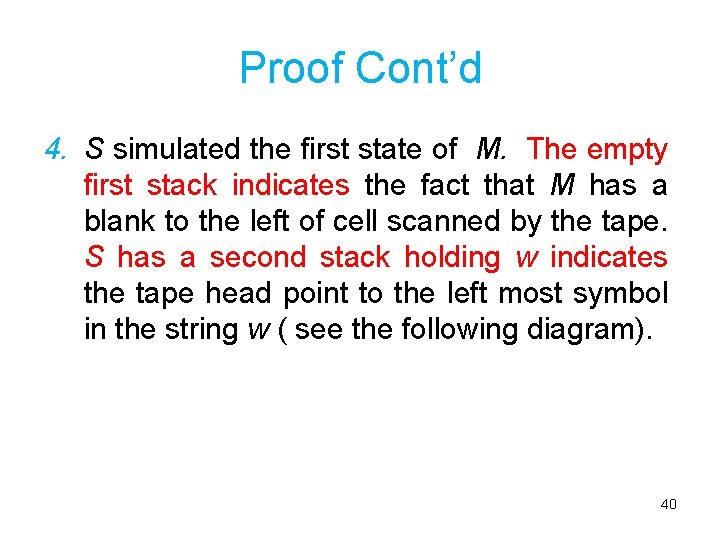Proof Cont’d 4. S simulated the first state of M. The empty first stack indicates the fact that M has a blank to the left of cell scanned by the tape. S has a second stack holding w indicates the tape head point to the left most symbol in the string w ( see the following diagram). 40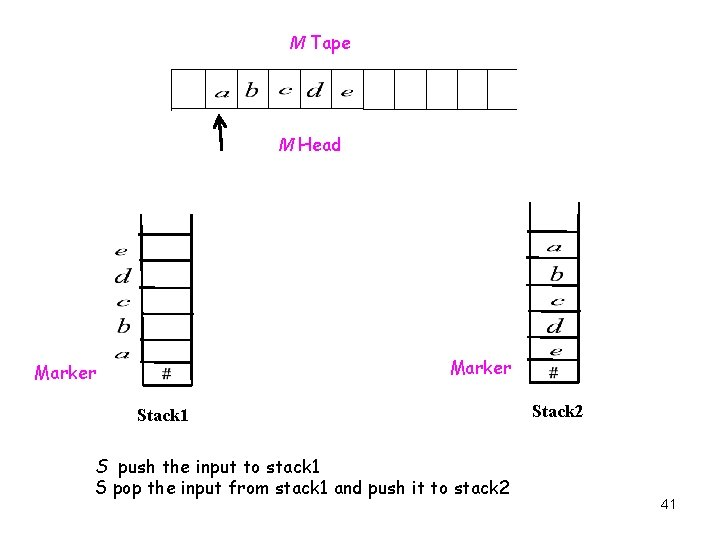M Tape M Head Marker Stack 1 S push the input to stack 1 S pop the input from stack 1 and push it to stack 2 Stack 2 41Proof Cont’d 5. S simulates a move of M as follows: a) S knows the state of M, say q, because S simulates the state of M in its own finite control. b) S knows the symbol X scanned by the head of M; it’s at the top of the second stack. If the second stack contains only the bottom of stack marker , this means that M’s head has just scanned a blank. 5. So, S knows the next move of M. 42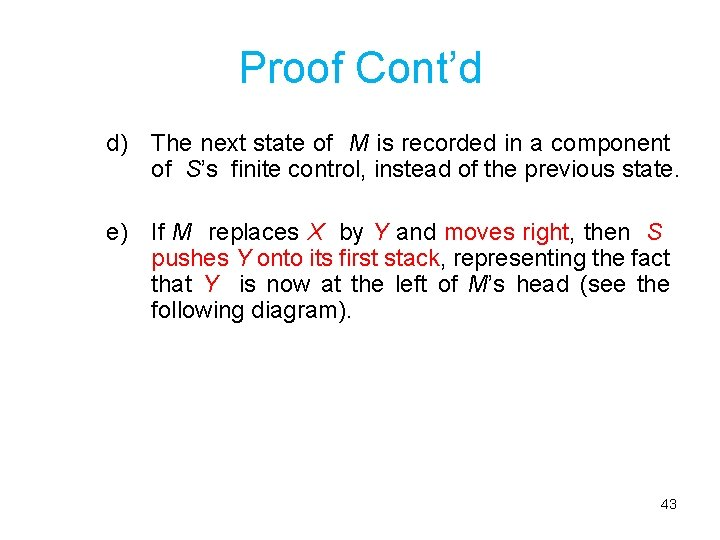Proof Cont’d d) The next state of M is recorded in a component of S’s finite control, instead of the previous state. e) If M replaces X by Y and moves right, then S pushes Y onto its first stack, representing the fact that Y is now at the left of M’s head (see the following diagram). 43M replaces X by Y and moves right M Tape Move right Pop Push Stack 1 S pop X from stack 2 and push Y to stack 1 Stack 2 44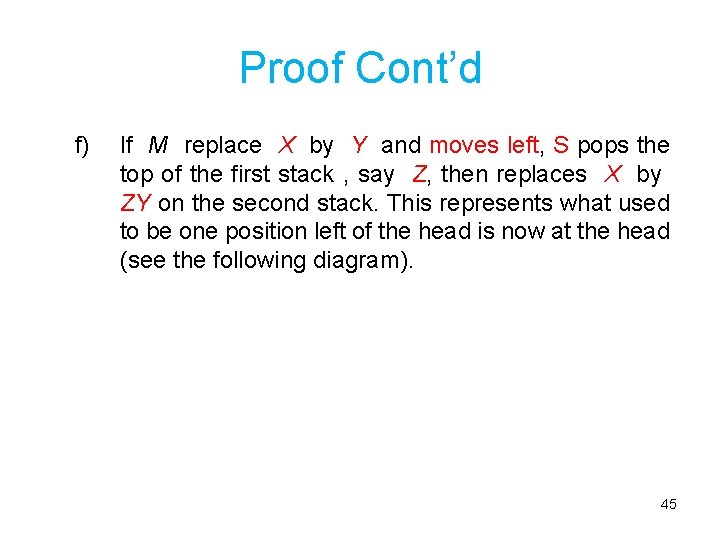Proof Cont’d f) If M replace X by Y and moves left, S pops the top of the first stack , say Z, then replaces X by ZY on the second stack. This represents what used to be one position left of the head is now at the head (see the following diagram). 45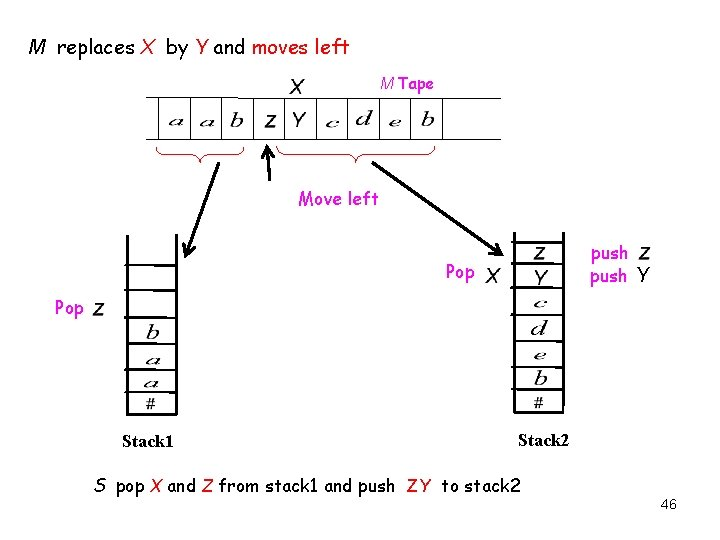M replaces X by Y and moves left M Tape Move left push Y Pop Stack 1 Stack 2 S pop X and Z from stack 1 and push ZY to stack 2 46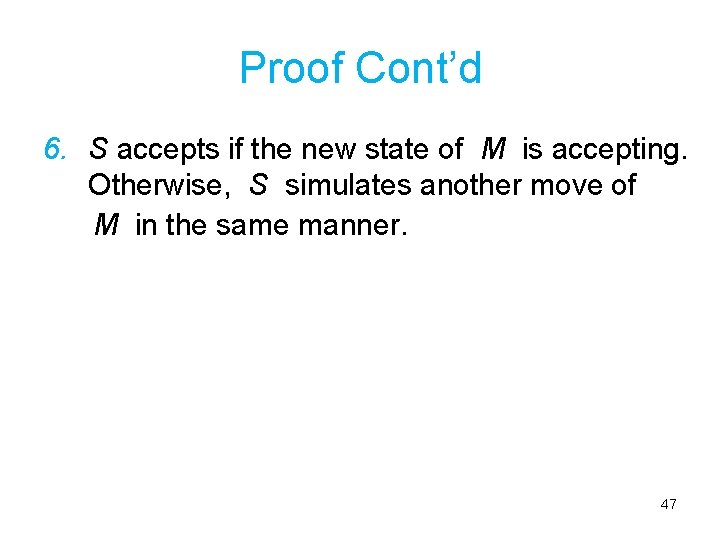Proof Cont’d 6. S accepts if the new state of M is accepting. Otherwise, S simulates another move of M in the same manner. 47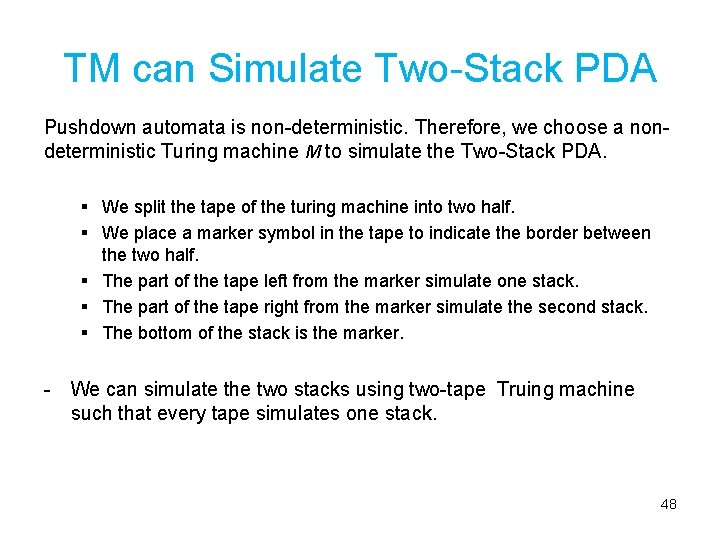TM can Simulate Two-Stack PDA Pushdown automata is non-deterministic. Therefore, we choose a nondeterministic Turing machine M to simulate the Two-Stack PDA. § We split the tape of the turing machine into two half. § We place a marker symbol in the tape to indicate the border between the two half. § The part of the tape left from the marker simulate one stack. § The part of the tape right from the marker simulate the second stack. § The bottom of the stack is the marker. - We can simulate the two stacks using two-tape Truing machine such that every tape simulates one stack. 48M Tape Marker indicates the two border of the tape Stack 1 Stack 2 49Question : Does the Two-Stack PDA simulate the computer? Answer: yes Since TM simulates a computer (proven in the course text book) AND Two-Stack PDA simulates a TM (proven in Hopcroft and Ullman ) Thus , Two-Stack PDA can simulate the computer. 50References 1 - Hopcroft J , Motwani R, Ullman J , “Introduction to Automata Theory, Languages and Computation”, Addison Wesley, 2 nd edition , 2001. 2 - T. A. Sudkamp , “Language and Machine: An Introduction to the Theory of Computer Science” Addison-Wesley , 2 nd edition , 1997. 51